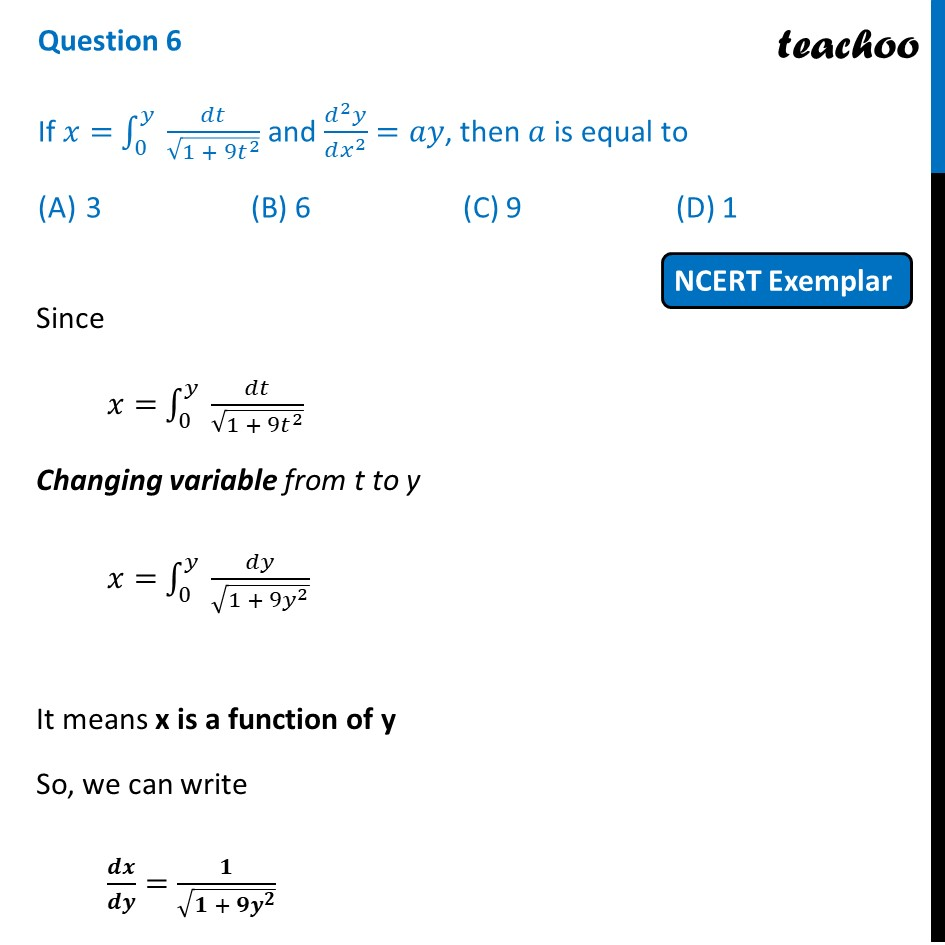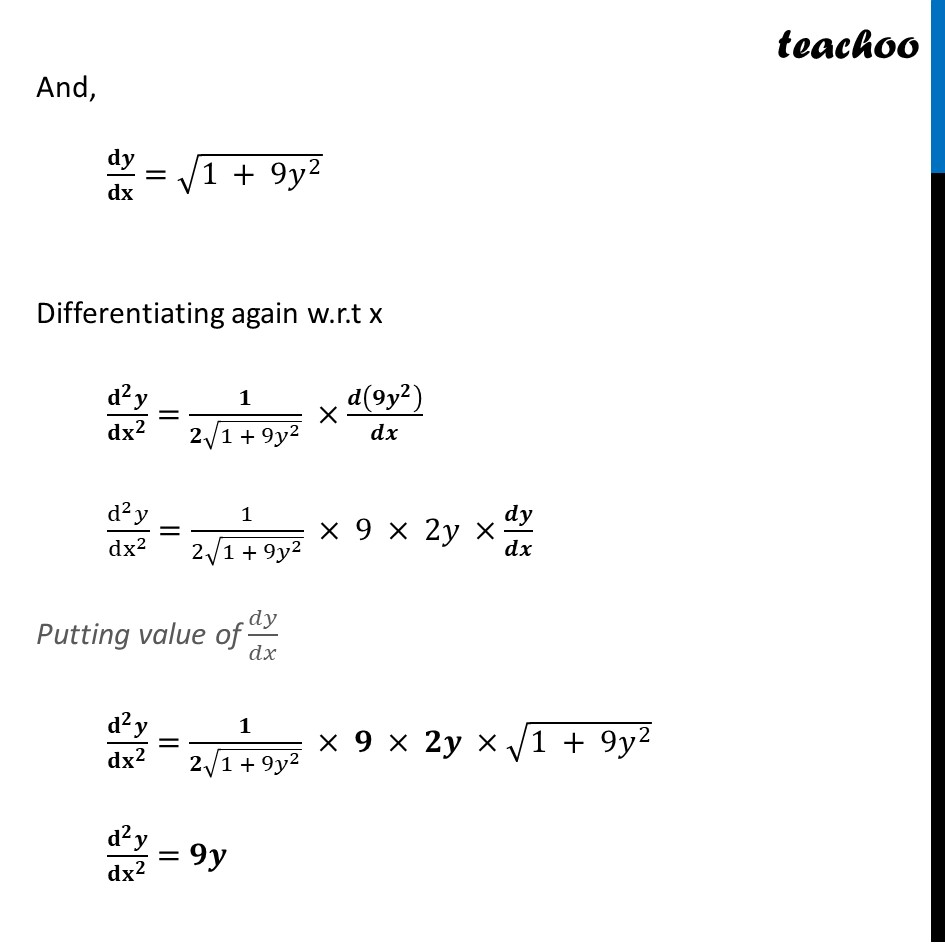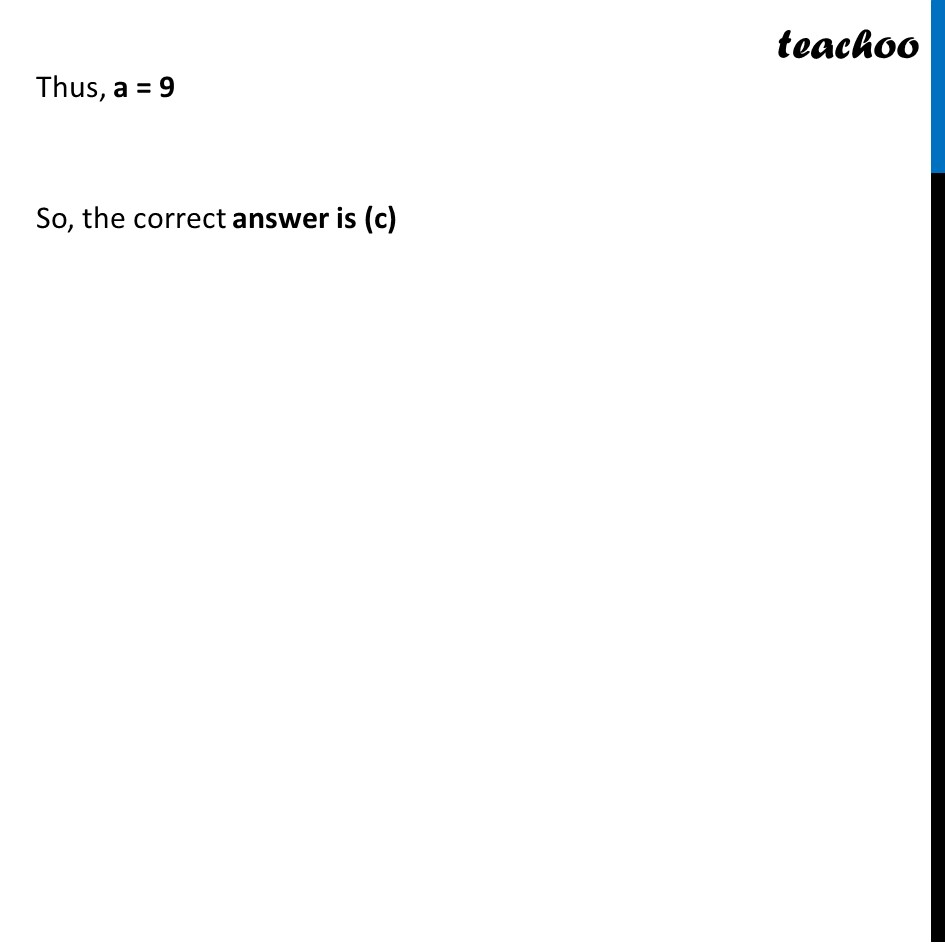NCERT Exemplar MCQ

Chapter 7 Class 12 Integrals
Serial order wise

## (D) 1Learn in your speed, with individual attention - Teachoo Maths 1-on-1 Class

### Transcript

Question 6 If 𝑥= ∫1_0^𝑦▒〖 𝑑𝑡/√(1 + 9𝑡^2 )〗 and (𝑑^2 𝑦)/(𝑑𝑥^2 )=𝑎𝑦, then 𝑎 is equal to 3 (B) 6 (C) 9 (D) 1 Since 𝑥= ∫1_0^𝑦▒〖 𝑑𝑡/√(1 + 9𝑡^2 )〗 Changing variable from t to y 𝑥= ∫1_0^𝑦▒〖 𝑑𝑦/√(1 + 9𝑦^2 )〗 It means x is a function of y So, we can write 𝒅𝒙/𝒅𝒚=𝟏/√(𝟏 + 𝟗𝒚^𝟐 ) And, 𝐝𝒚/𝐝𝐱=√(1 + 9𝑦^2 ) Differentiating again w.r.t x (𝐝^𝟐 𝒚)/(𝐝𝐱^𝟐 )=𝟏/(𝟐√(1 + 9𝑦^2 )) ×𝒅(𝟗𝒚^𝟐 )/𝒅𝒙 (d^2 𝑦)/(dx^2 )=1/(2√(1 + 9𝑦^2 )) × 9 × 2𝑦 ×𝒅𝒚/𝒅𝒙 Putting value of 𝑑𝑦/𝑑𝑥 (𝐝^𝟐 𝒚)/(𝐝𝐱^𝟐 )=𝟏/(𝟐√(1 + 9𝑦^2 )) × 𝟗 × 𝟐𝒚 ×√(1 + 9𝑦^2 ) (𝐝^𝟐 𝒚)/(𝐝𝐱^𝟐 )=𝟗𝒚 Thus, a = 9 So, the correct answer is (c)